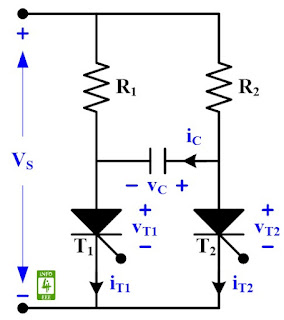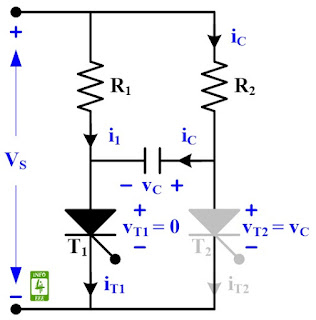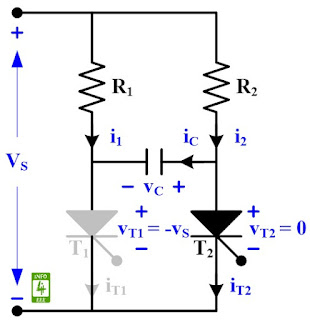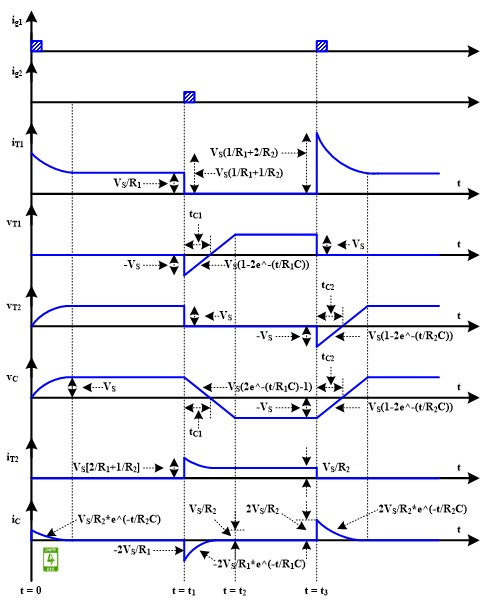Complementary commutation is also called voltage commutation, forced commutation, complimentary impulse commutation, or Class-C commutation. It is used in dc choppers and inverters. A thyristor carrying load current is commutated by transferring its load current to another incoming thyristor. i.e. Firing of T1 commutates T2 and firing of T2 commutates T1. A circuit diagram of the complementary commutation technique of thyristor is given in Fig. 1.Fig. 1 Circuit diagram of complementary commutation.
At t = 0, T1 is turned ON and iT1 = i1 + iC = v ( 1/R1 + 1/R2 ). The capacitor is initially uncharged so vC = 0, iC(t) = VS/R2*e^(-t/R2C) and vC(t) = vT2 = VS(1-e^(-t/R2C).Fig. 2 Circuit diagram of complementary commutation at t = 0.
At t = t1, T2 is turned ON, and -vC is applied across T1 to turn it OFF. So vT2 = 0, vT1 = -VS, iC = -2VS/R1, and iT2 = VS(1/R1+1/R2). By KVL, R1*i+ 1/C * ∫idt = VS. Aftter taking Laplace, R1*IC(s) + 1/C*[IC(s)/s - C*VS/s] = VS/s. iC(t) = 2VS/R1*e^(-t/R1C). As iC(t) flows in opposite direction, iC(t) = -2VS/R1*e^(-t/R1C). vC(t) = [1/C*∫idt + VS] = [1/C*∫(-2VS/R1*e^(-t/R1C + VS)] = VS[2e^(-t/R1C) - 1]. Note: Integration is done from 0 to t.Fig. 3 Circuit diagram of complementary commutation at t = t1.
At t = t3, T1 is turned ON, iT2 = 0, iT1 = V[2/R+ 1/R1), vT2 = -VS, vT1 = 0, iC = 2*VS/R2. VS applies a sudden reverse bias across T2 to turn it OFF. vT1 = 0 = VS(1-2e^(-tc1/R1C). tc1 = R1C*ln(2) and tc2 = R2C*ln(2). Where tc1 is circuit turn OFF time for T1.Fig. 4 Waveform of complementary commutation

#### Reference

Dr. P. S. Bimbhra, "Power Electronics", Khanna Publishers, Fifth Edition, 2013.

#### Author

authored 77 articles for INFO4EEE Website since 2012.